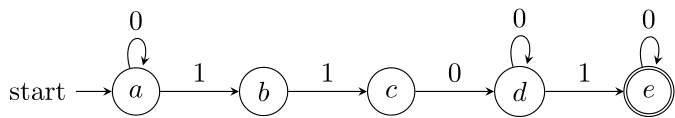Constraint regular

The constraint regular, see [CB04] and [P04], ensures that the sequence of values assigned to the variables it involves forms a word that can be recognized by a deterministic (or non-deterministic) finite automaton.

regular($X$,$A$) with $X=\langle x_1,x_2,\ldots,x_r \rangle$ and $A$ a finite automaton , iff $x_1x_2 \ldots x_r \in L(A)$ where $L(A)$ denotes the language recognized by the deterministic (or non-deterministic) finite automaton $A$.

The syntax for regular is:

Syntax

<regular>
<list> (intVar wspace)+ </list>
<transitions> ("(" state "," intVal "," state ")")+ </transitions>
<start> state </start>
<final> (state wspace)+ </final>
</regular>

As an example, let us consider the constraint defined on scope $\langle x_1,x_2,\ldots,x_7 \rangle$ from the simple automation depicted below:We obtain:

Example

<regular>
<list> x1 x2 x3 x4 x5 x6 x7 </list>
<transitions>
(a,0,a)(a,1,b)(b,1,c)(c,0,d)(d,0,d)(d,1,e)(e,0,e)
</transitions>
<start> a </start>
<final> e </final>
</regular>